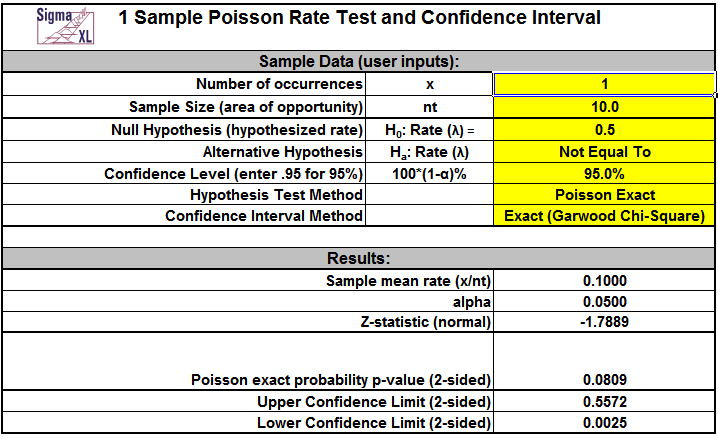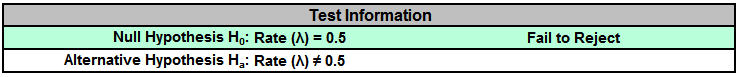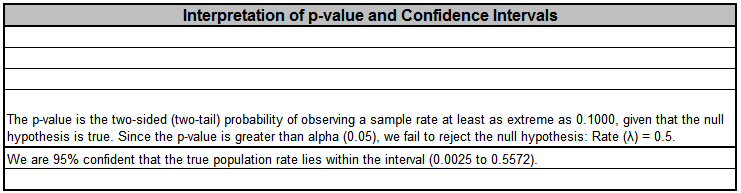Include Top

Basic Statistical Templates - 1 Poisson Rate Test and Confidence Interval Example

Click SigmaXL > Templates & Calculators > Basic Statistical Templates >1 Poisson Rate Test and Confidence Interval to access the template.Notes

1. Enter summarized Sample Data, Null Hypothesis and Confidence Level in cells with yellow highlight. Do not modify any other part of this worksheet.
2. Sample Size (area of opportunity) can be a count, time, length or other unit that defines the area of opportunity for an occurrence.
3. Select Alternative Hypothesis and Methods using drop-down. If x = 0, it is recommended that the Alternative Hypothesis be set to one-sided "Less Than."
4. Hypothesis Test Method:
• Poisson Exact is recommended.
• Use Normal Approximation only when the number of occurrences, x > 10.
5. Confidence Interval Method (recommendations based on literature review, see references below):
• Confidence intervals for Poisson rates have an "oscillation" phenomenon where the coverage probability varies with x and n.
• Exact (Garwood Chi-Square) is strictly conservative and will guarantee the specified confidence level as a minimum coverage probability, but results in wide intervals. Recommended only for applications requiring strictly conservative intervals.
• Jeffreys has mean coverage probability matching the specified confidence interval and is recommended.
• Normal Approximation (Wald) is not recommended, but included here to validate hand calculations. Use only when the number of occurrences, x > 10.
6. This calculator will automatically detect the version of Excel and use the appropriate statistical functions.

## References:

Garwood, F. (1936). Fiducial limits for the Poisson distribution. Biometrika 28:437–442.

Swift, M.B. (2009). Comparison of confidence intervals for a Poisson Mean-Further considerations, Communication in Statistics — Theory and Methods, 38, 748–759.

# Web Demos

Our CTO and Co-Founder, John Noguera, regularly hosts free Web Demos featuring SigmaXL and DiscoverSim